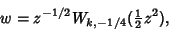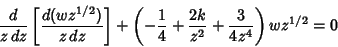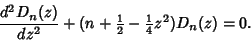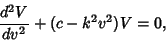## Weber Differential Equations

Consider the differential equation satisfied by(1)

whereis a Whittaker Function.(2)(3)

This is usually rewritten(4)

The solutions are Parabolic Cylinder Functions.

The equations(5)(6)

which arise by separating variables in Laplace's Equation in Parabolic Cylindrical Coordinates, are also known as the Weber differential equations. As above, the solutions are known as Parabolic Cylinder Functions.